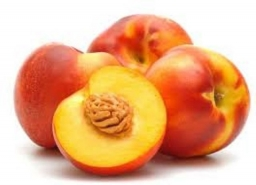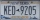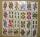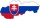# Peaches

There are 20 peaches in the pocket. 3 peaches are rotten. What is the probability that one of the randomly picked two peaches will be just one rotten?

p =  0.2684

### Step-by-step explanation:Did you find an error or inaccuracy? Feel free to write us. Thank you!Tips to related online calculators
Would you like to compute count of combinations?

## Related math problems and questions:

• White and black ballsThere are 7 white and 3 black balls in an opaque pocket. The balls are the same size. a) Randomly pull out one ball. What is the probability that it will be white? We pull out one ball, see what color it is and return it to the pocket. Then we pull out th
• ClassroomOf the 26 pupils in the classroom, 12 boys and 14 girls, four representatives are picked to the odds of being: a) all the girls b) three girls and one boy c) there will be at least two boys
• Boys and girlsThere are 11 boys and 18 girls in the classroom. Three pupils will answer. What is the probability that two boys will be among them?A license plate has 3 letters followed by 4 numbers. Repeats are not allowed for the letters, but they are for the numbers. If they are issued at random, what is the probability that the 3 letters are in alphabetical order and the 3 numbers are consecutiv
• CardsFrom a set of 32 cards, we randomly pull out three cards. What is the probability that it will be seven kings and ace?
• Boys and girlsThere are eight boys and nine girls in the class. There were six children on the trip from this class. What is the probability that left a) only boys b) just two boys
• Sum or productWhat is the probability that two dice fall will have the sum 7 or product 12?
• Weekly serviceIn the class are 20 pupils. How many opportunities have the teacher selected two pupils who will have a week-class service randomly?
• Two acesFrom a 32 card box we randomly pick 1 card and then 2 more cards. What is the probability that last two drawn cards are aces?
• One threeWe throw two dice. What is the probability that max one three falls?
• BallsThe urn is 8 white and 6 black balls. We pull 4 randomly balls. What is the probability that among them will be two white?
• Family94 boys are born per 100 girls. Determine the probability that there are two boys in a randomly selected family with three children.
• Families 2Seven hundred twenty-nine families are having six children each. The probability of a girl is 1/3, and the probability of a boy is 2/3. Find the number of families having two girls and four boys.
• Three redsWhat is the probability that when choosing 3 carats from seven carats, all 3 reds will be red?
• School parliamentThere are 18 boys and 14 girls in the class. In how many ways can 3 representatives be elected to the school parliament, if these are to be: a) the boys themselves b) one boy and two girls
• Three digits numberFrom the numbers 1, 2, 3, 4, 5 create three-digit numbers that digits not repeat and number is divisible by 2. How many numbers are there?
• WordWhat is the probability that a random word composed of chars E, Y, G, E, R, O, M, T will be the GEOMETRY?